1.函数是什么

2.c语言中函数的分类：

2.1. 库函数

2. 自定义函数

3. 函数的参数

3.1 实际参数（实参）

3.2 形式参数（形参）

4. 函数的调用：

4.1 传值调用

4.2 传址调用

5. 函数的嵌套调用和链式访问

5.1 嵌套调用

5.2 链式访问

6.函数的声明和定义

6.1 函数声明：

6.2 函数定义：

7. 函数递归

7.1 什么是递归？

7.2 递归的两个必要条件

# 2.c语言中函数的分类：

## 2.1. 库函数

cplusplus.com - The C++ Resources Network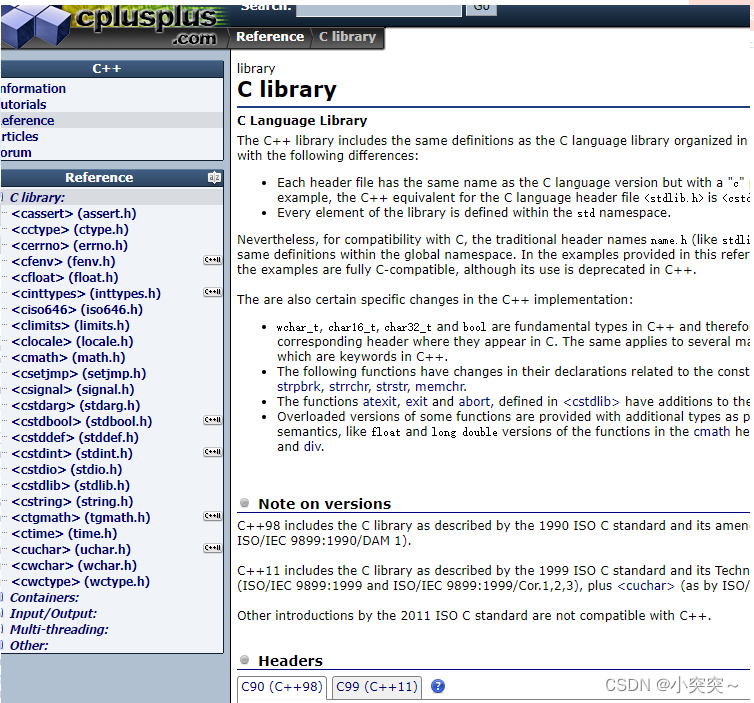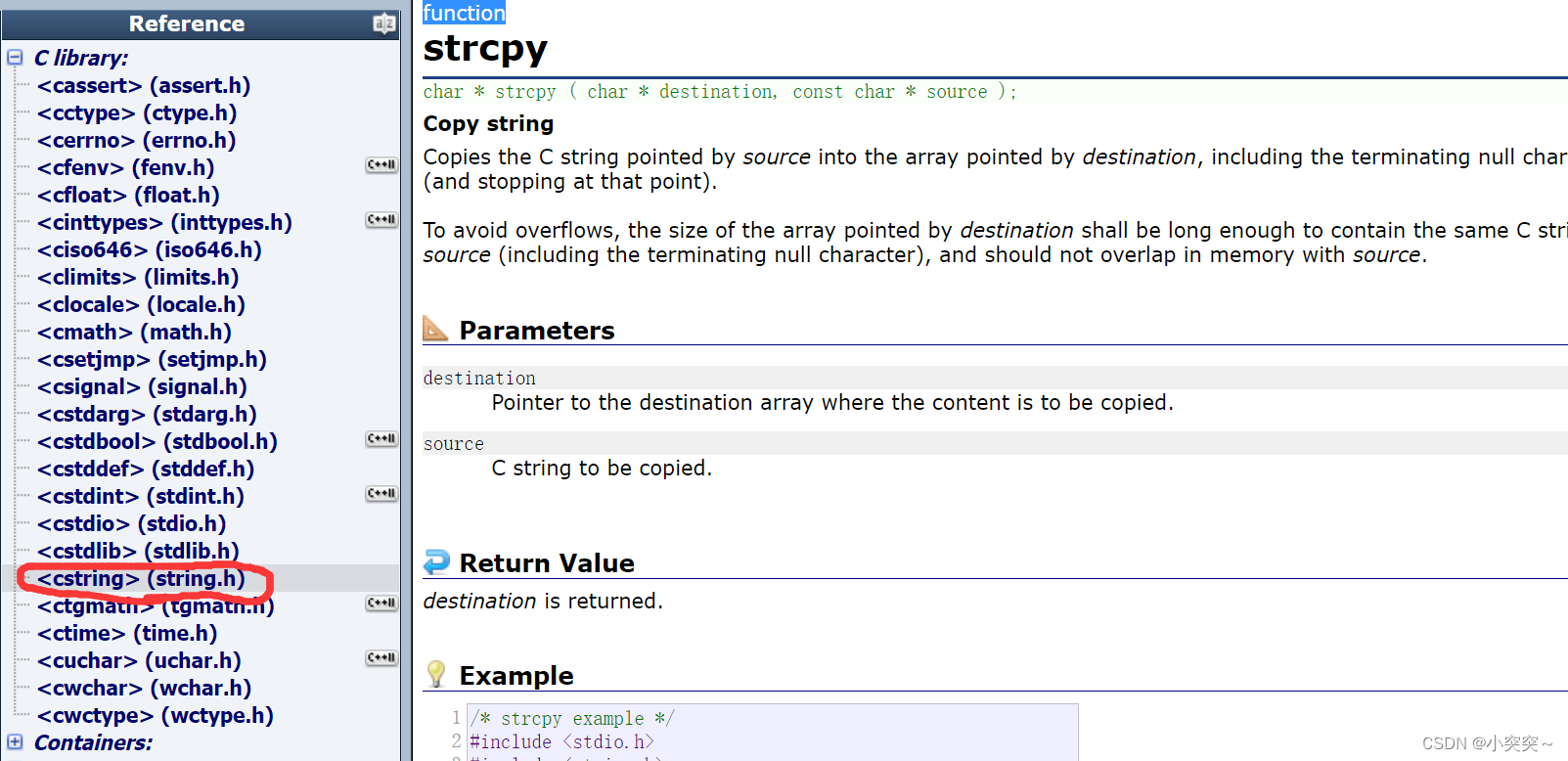strcpy这个函数包含在string.h这个头文件里面，这个函数的参数需要两个指针，返回值是一个字符型指针，指针就是地址，char * strcpy ( char * destination, const char * source );

Copies the C string pointed by source into the array pointed by destination, including the terminating null character (and stopping at that point).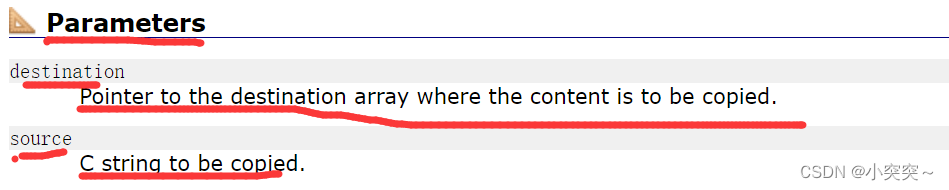Pointer to the destination array where the content is to be copied.

C string to be copied.返回值是destiination，destiination是一个字符型指针。

## 2. 自定义函数

ret_type fun_name(para1, * )
{
statement;//语句项
}
ret_type 返回类型
fun_name 函数名
para1   函数参数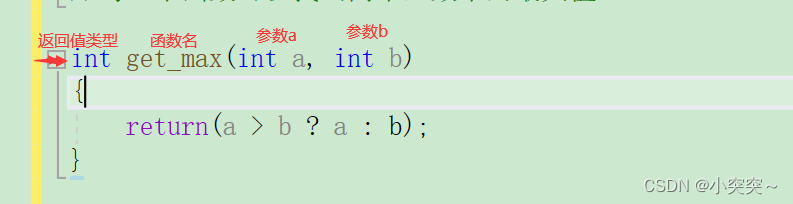# 3. 函数的参数

## 3.2 形式参数（形参）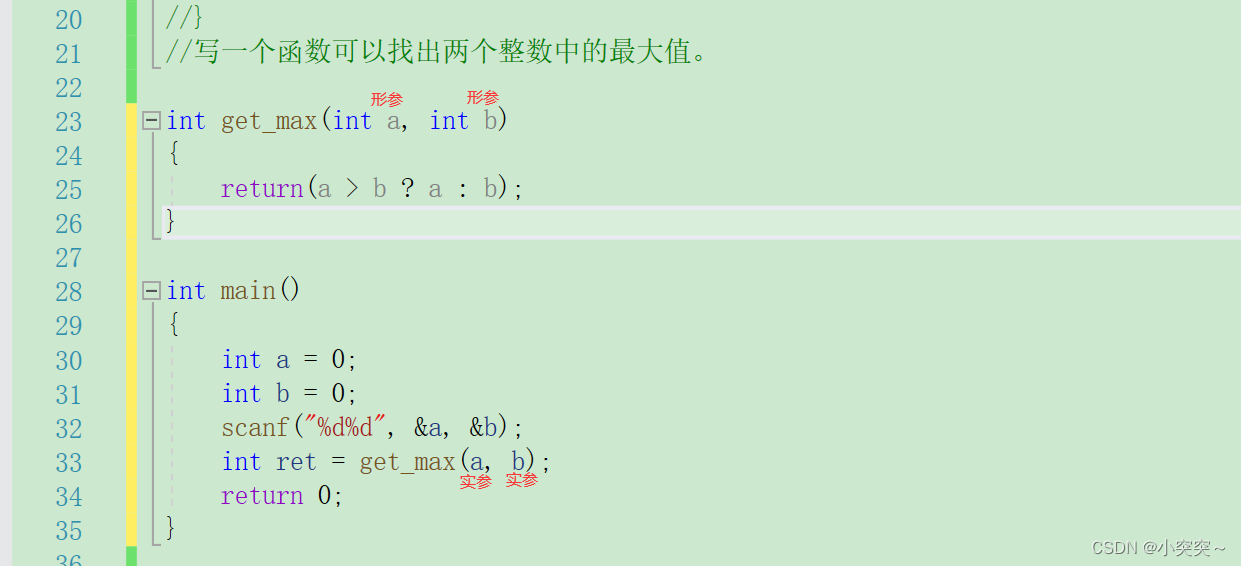# 4. 函数的调用：

## 4.1 传值调用

``````void swap(int p1, int p2)
{
int tmp = 0;
tmp = p1;
p1 = p2;
p2 = tmp;
}
int main()
{
int a = 0;
int b = 0;
scanf("%d%d", &a, &b);
printf("交换前，a = %d b = %d\n", a, b);
swap(a, b);
/*int p1 = &a;
int p2 = &b;
swap(p1, p2);*/
printf("交换后，a = %d b = %d\n", a, b);
return 0;
}``````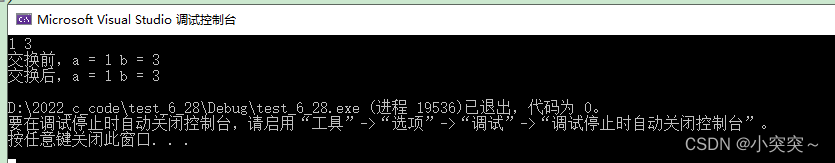## 4.2 传址调用

``````void swap(int* p1 , int* p2)
{
int tmp = 0;
tmp = *p1;
*p1 = *p2;
*p2 = tmp;
}
int main()
{
int a = 0;
int b = 0;
scanf("%d%d", &a, &b);
printf("交换前，a = %d b = %d\n", a, b);
swap(&a, &b);
/*int p1 = &a;
int p2 = &b;
swap(p1, p2);*/
printf("交换后，a = %d b = %d\n", a, b);
return 0;
}``````

# 5. 函数的嵌套调用和链式访问

## 5.1 嵌套调用

``````#include <stdio.h>
void new_line()
{
printf("hehe\n");
}
void three_line()
{
int i = 0;
for (i = 0; i < 3; i++)
{
new_line();
}
}
int main()
{
three_line();
return 0;
}``````
``````int main()
{
void test()
{
//......
}
return 0;
}``````

这种就是嵌套定义，即在一个函数内部去定义函数，是不可以的。

## 5.2 链式访问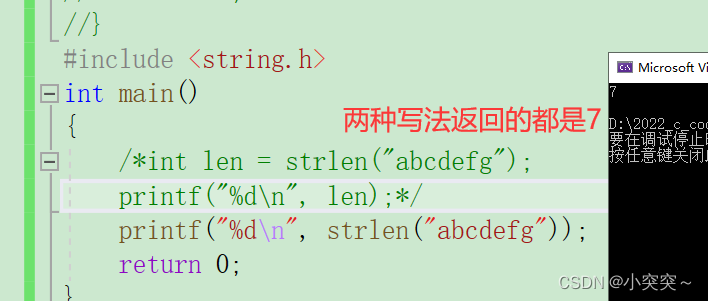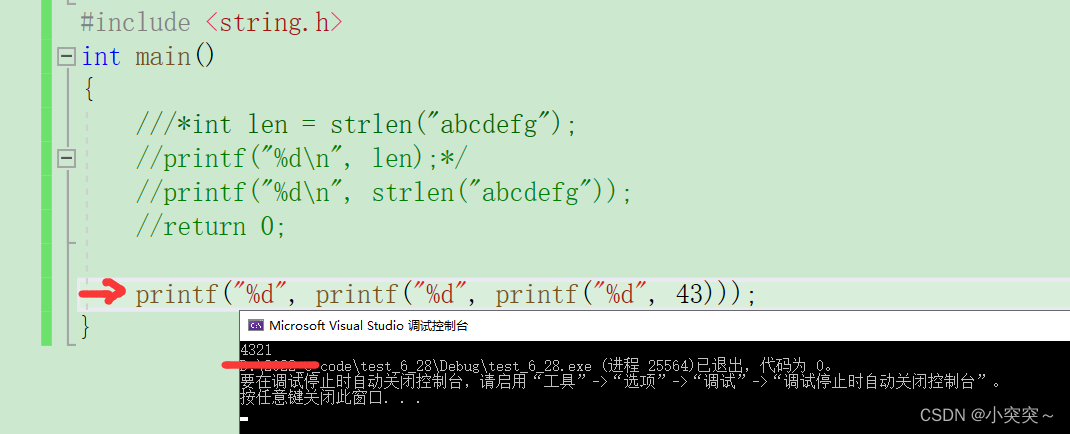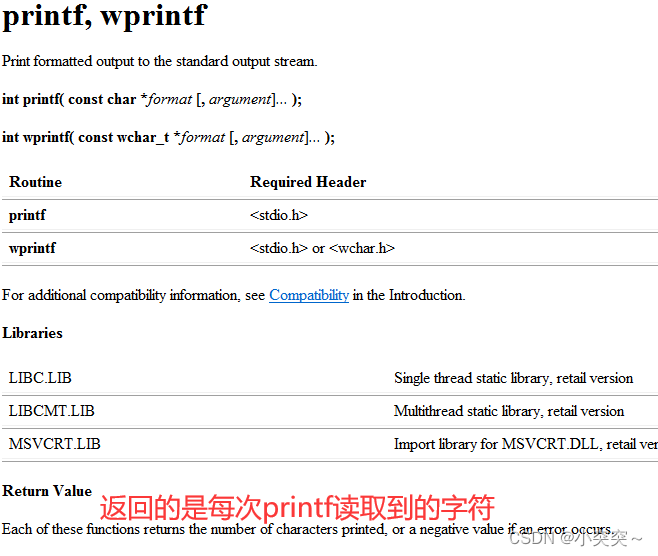# 6.函数的声明和定义

## 6.1 函数声明：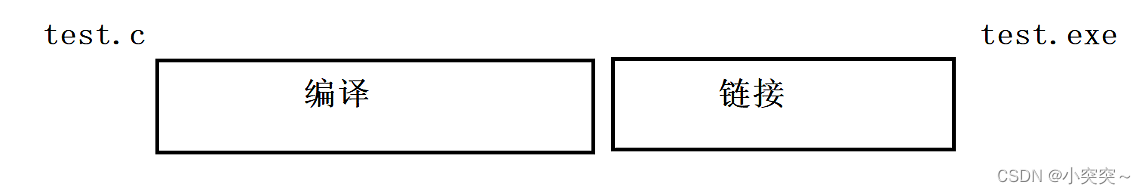程序在执行的时候是一步一步往下走的，下面这张图则是教科书上的写法，我们调用一个函数时，要有函数的声明，可如果函数写在了main函数的上方，则不余需要声明了。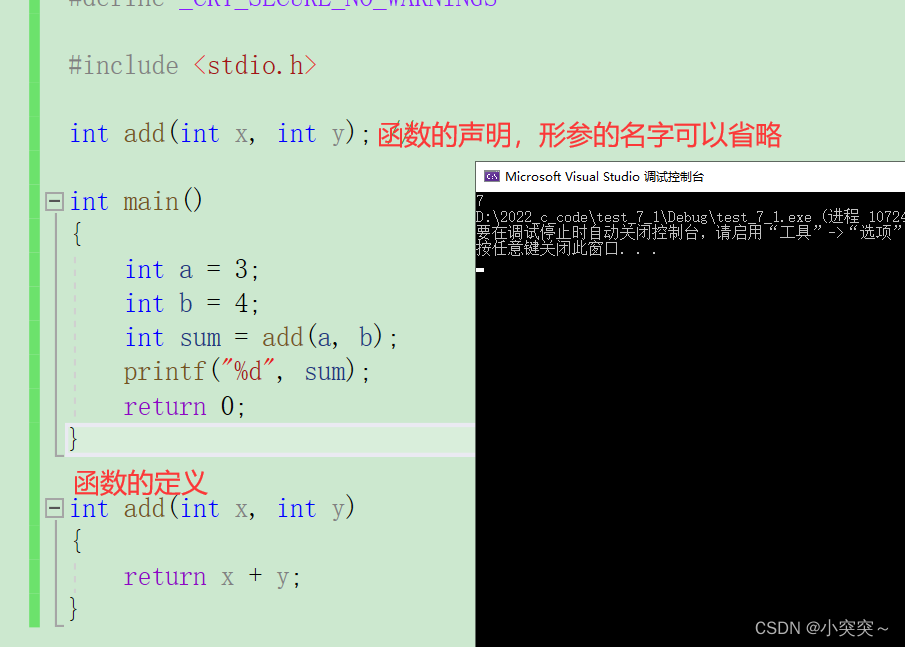1. 告诉编译器有一个函数叫什么，参数是什么，返回类型是什么。但是具体是不是存在，函数
声明决定不了。
2. 函数的声明一般出现在函数的使用之前。要满足先声明后使用。
3. 函数的声明一般要放在头文件中的。

## 6.2 函数定义：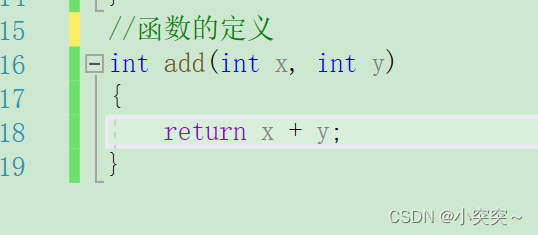# 6.3 工作中的写法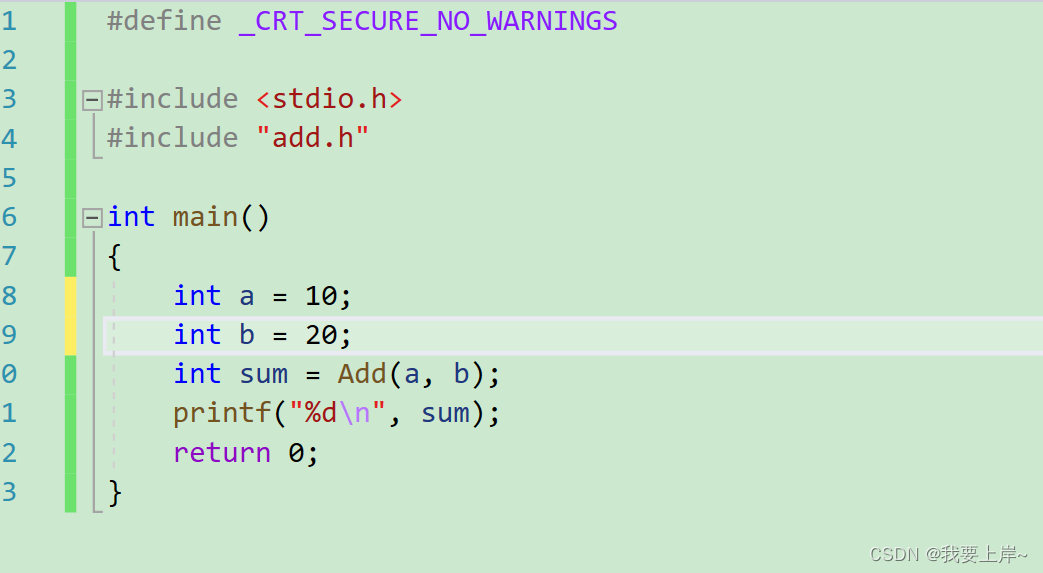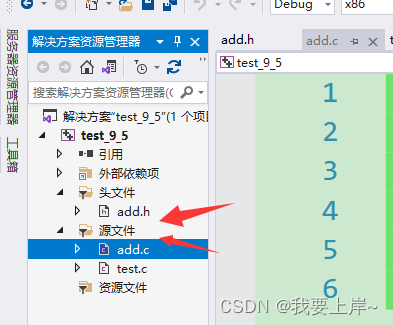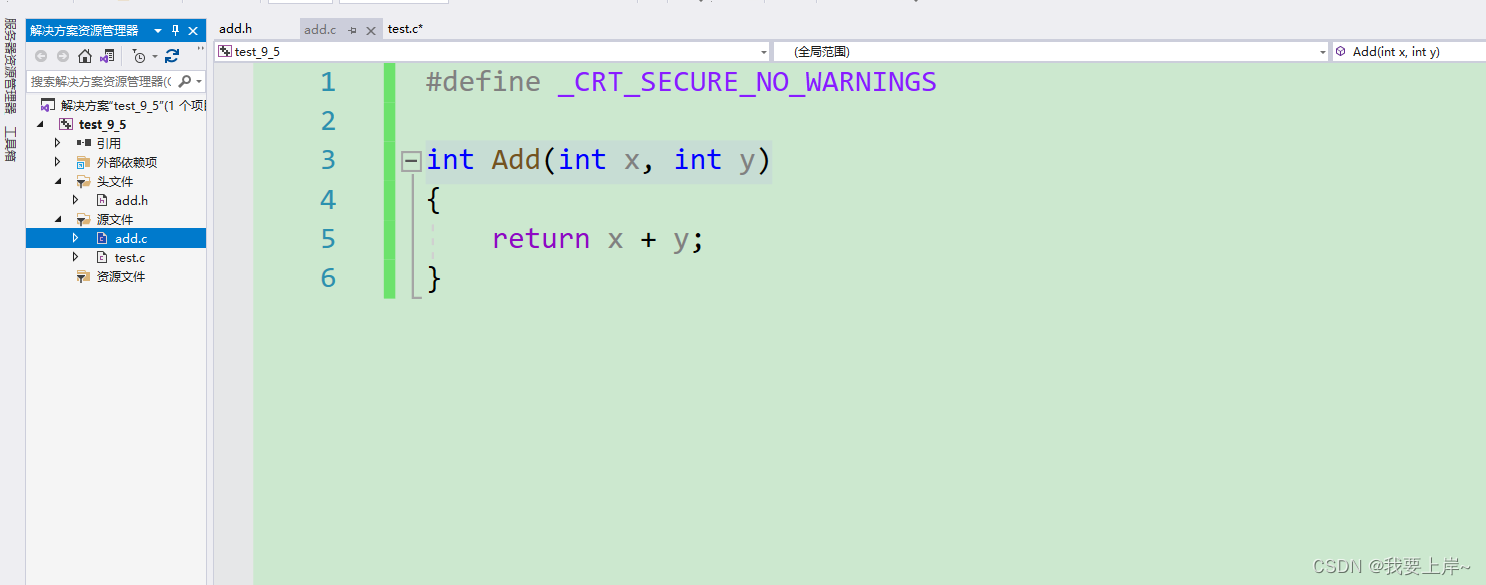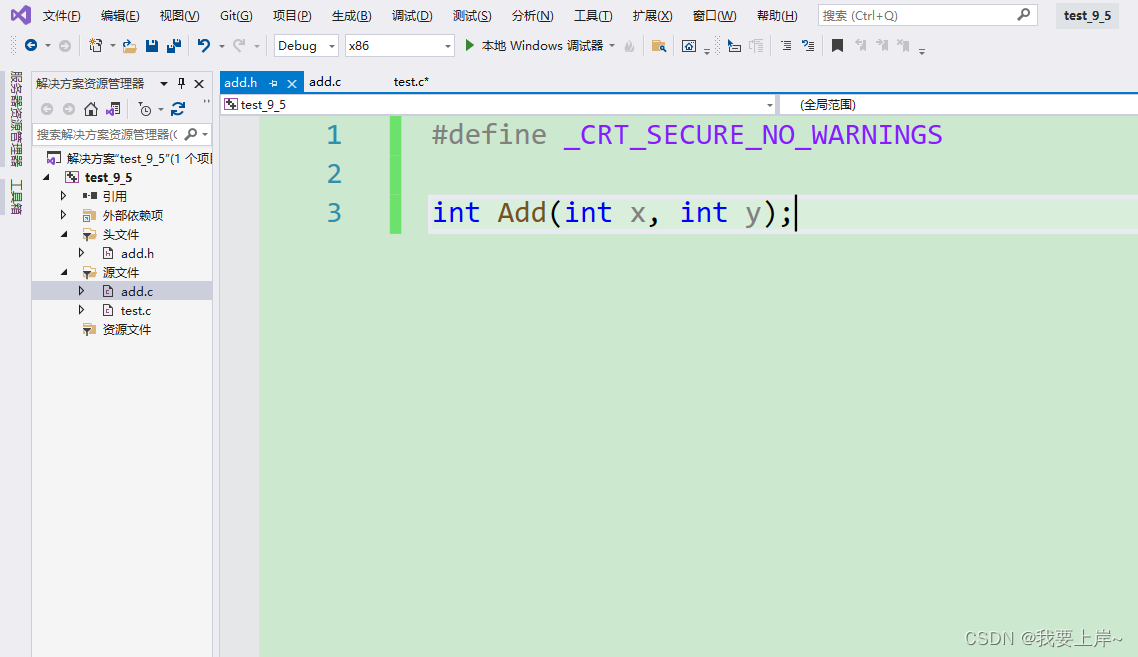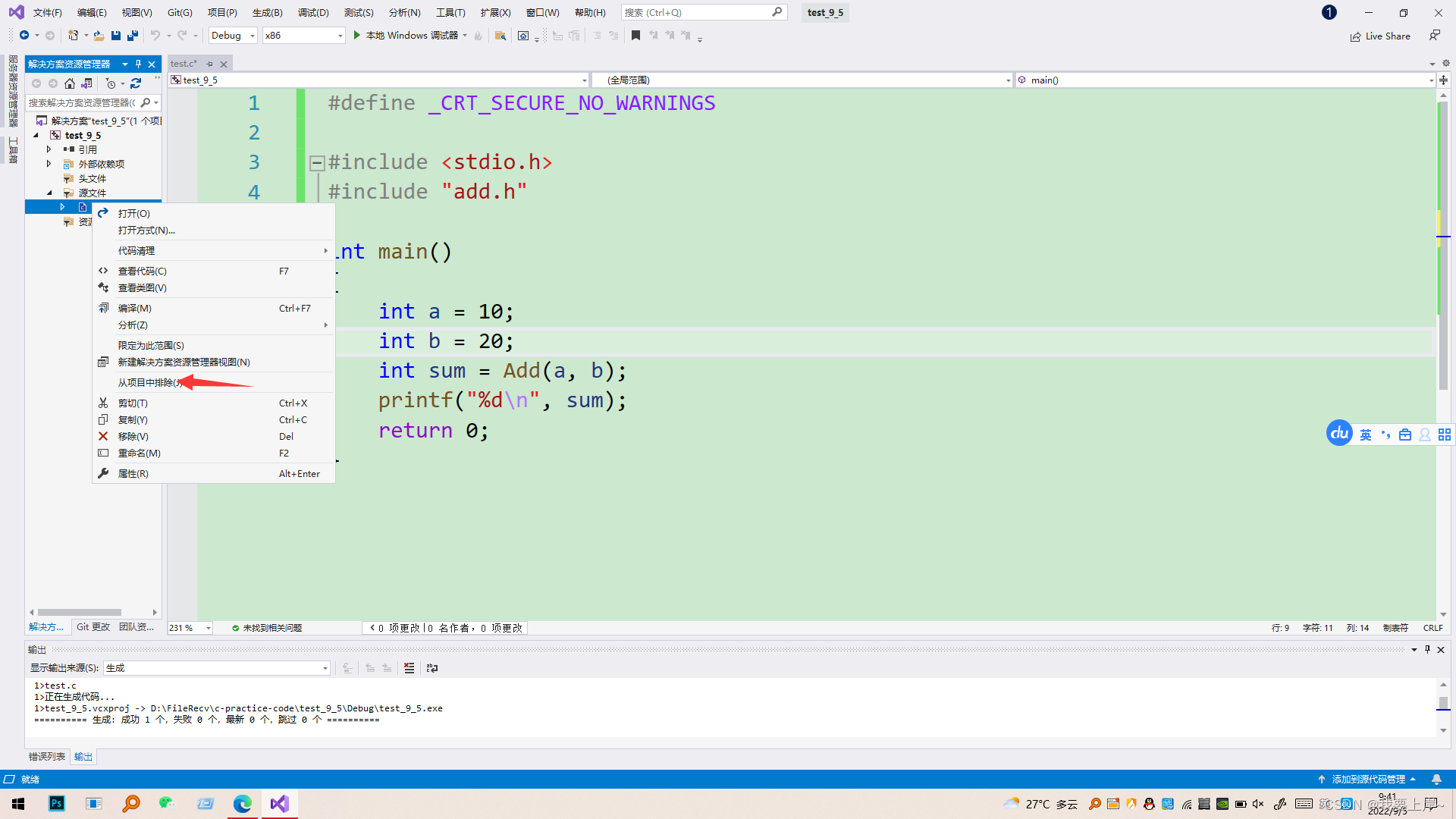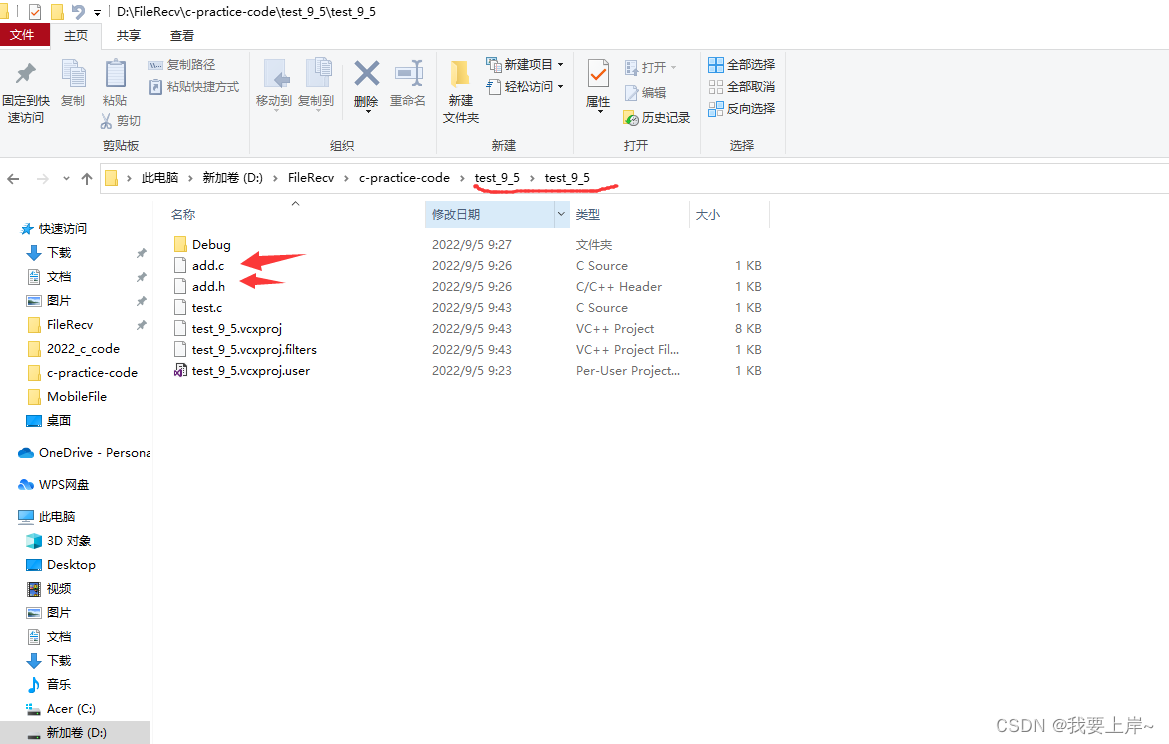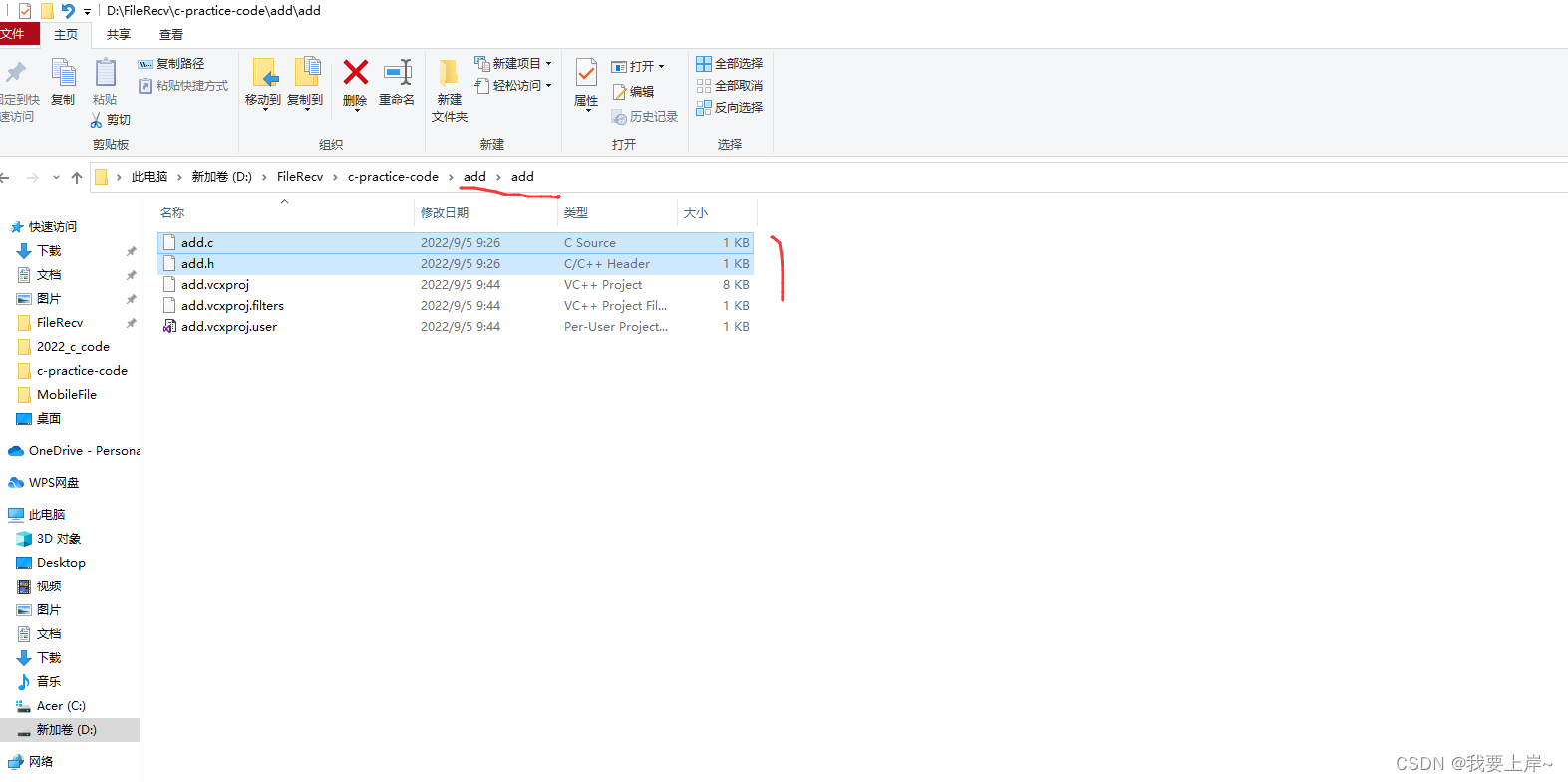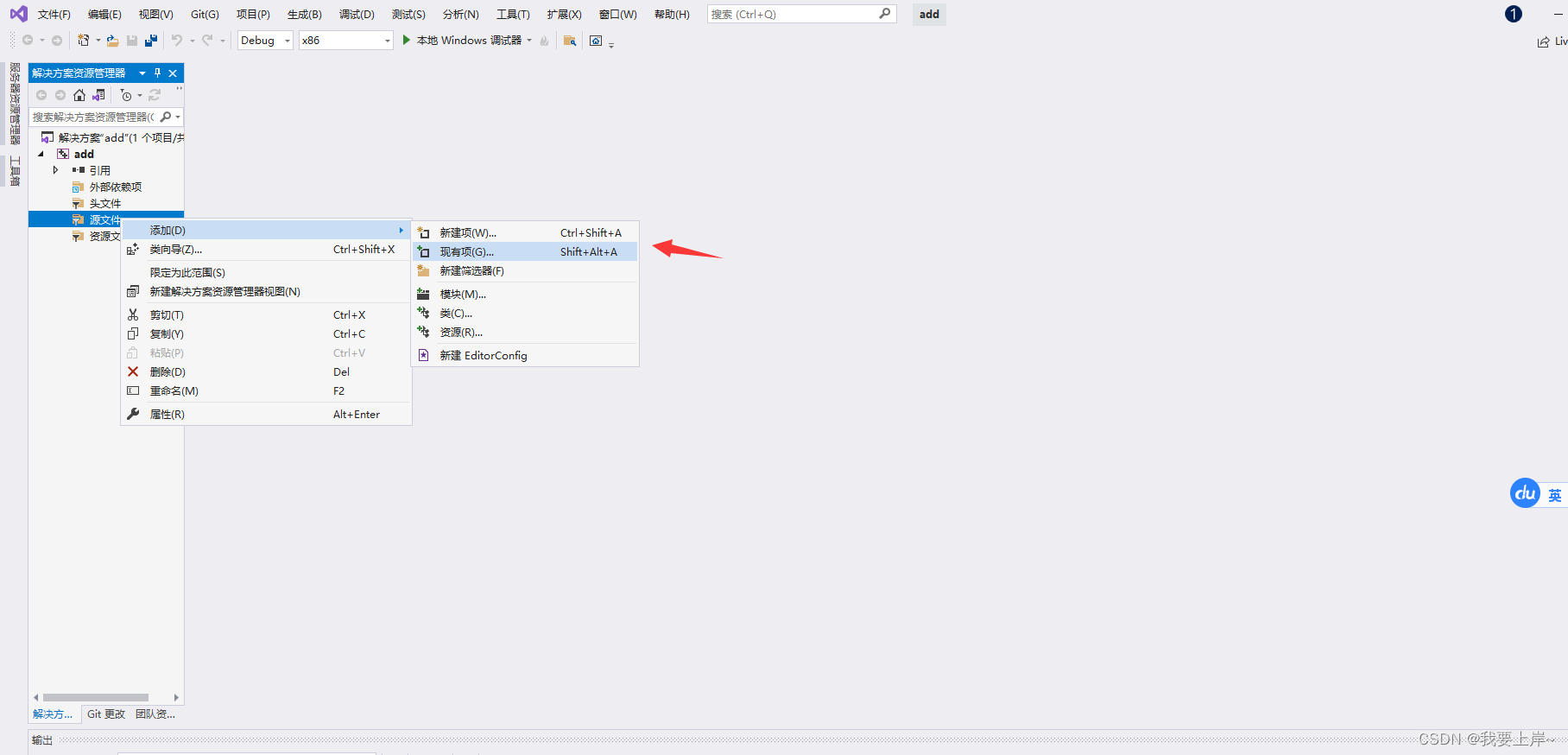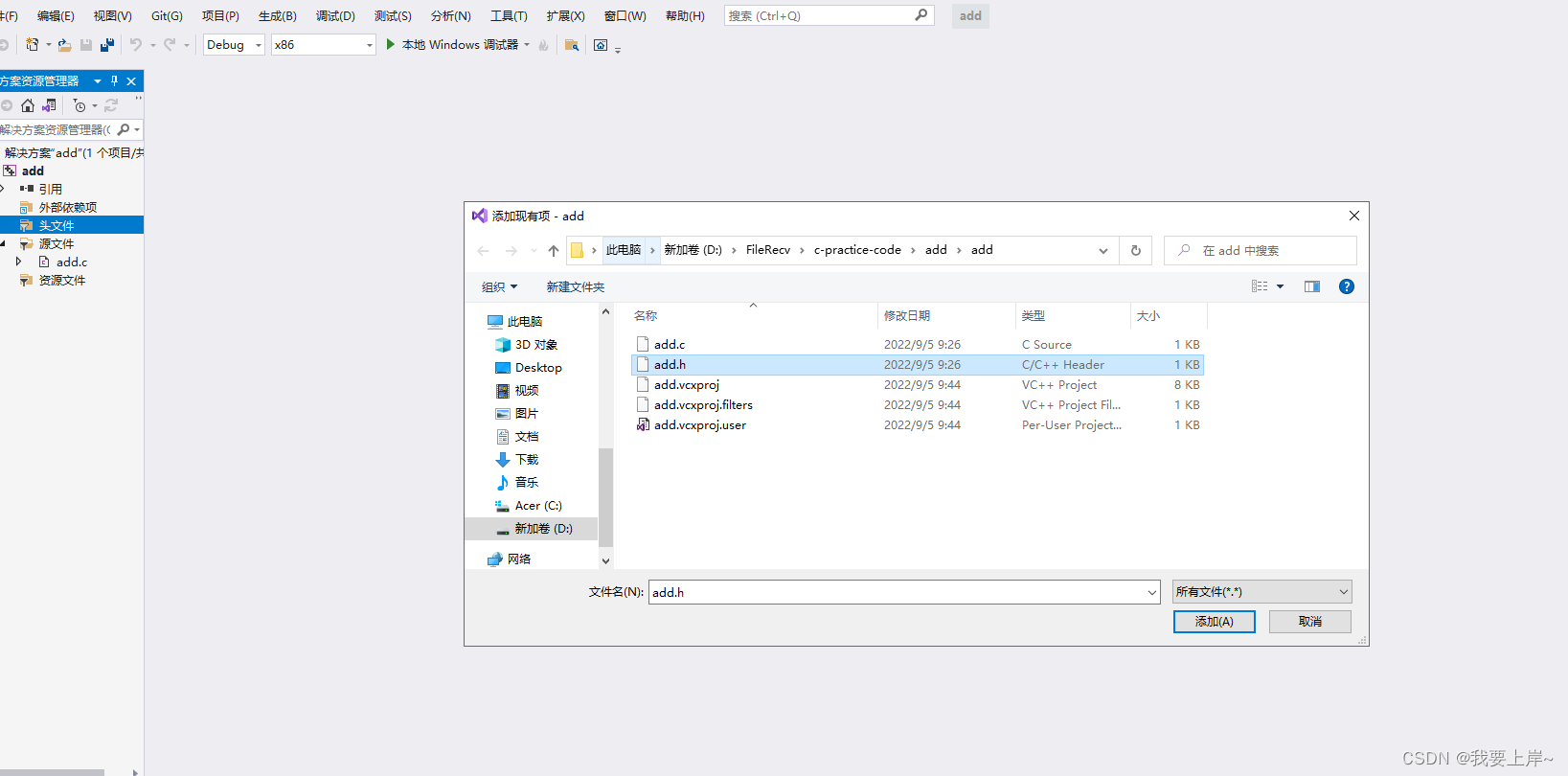如果，不愿意暴露代码。可以编译成静态库。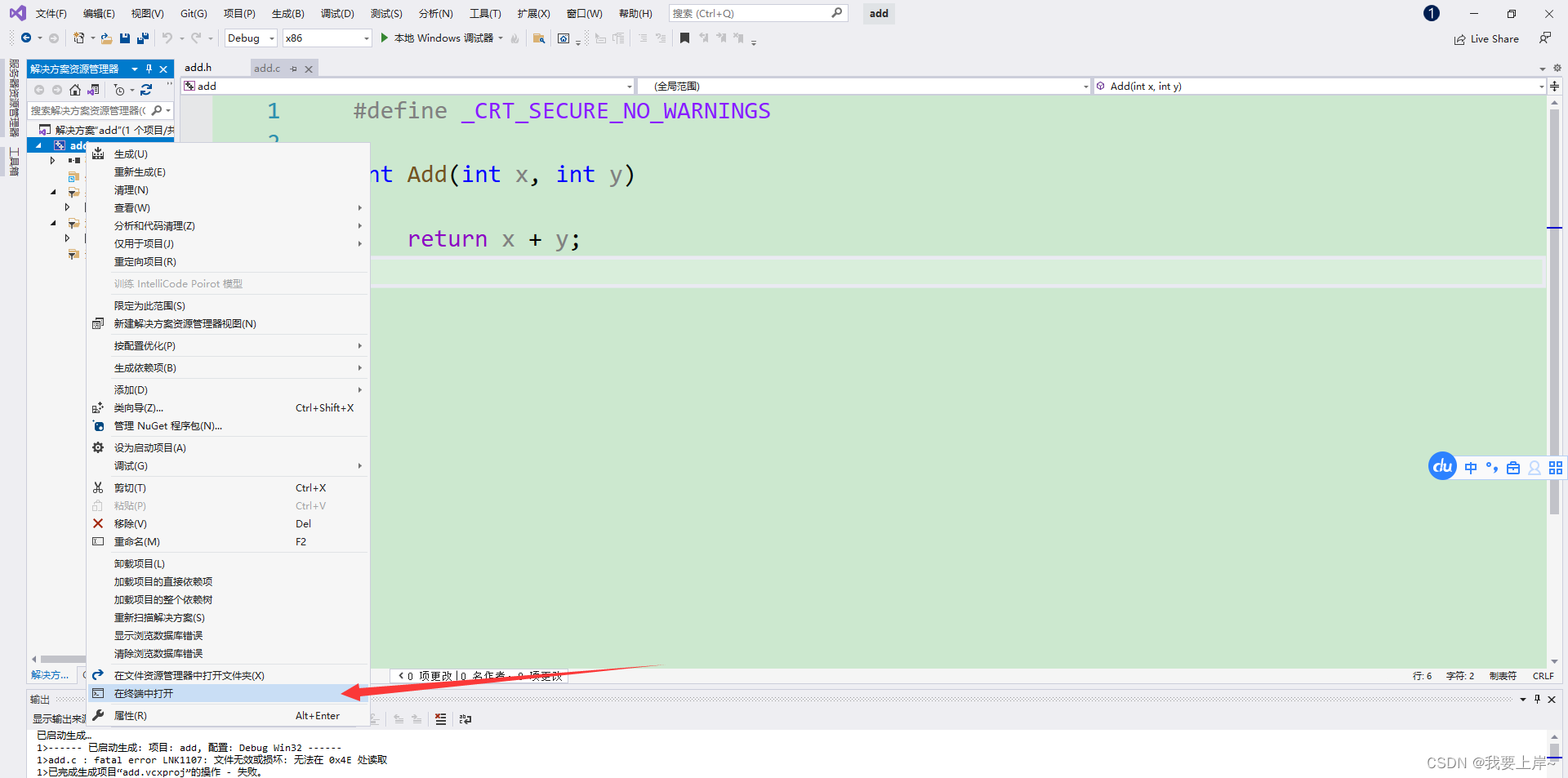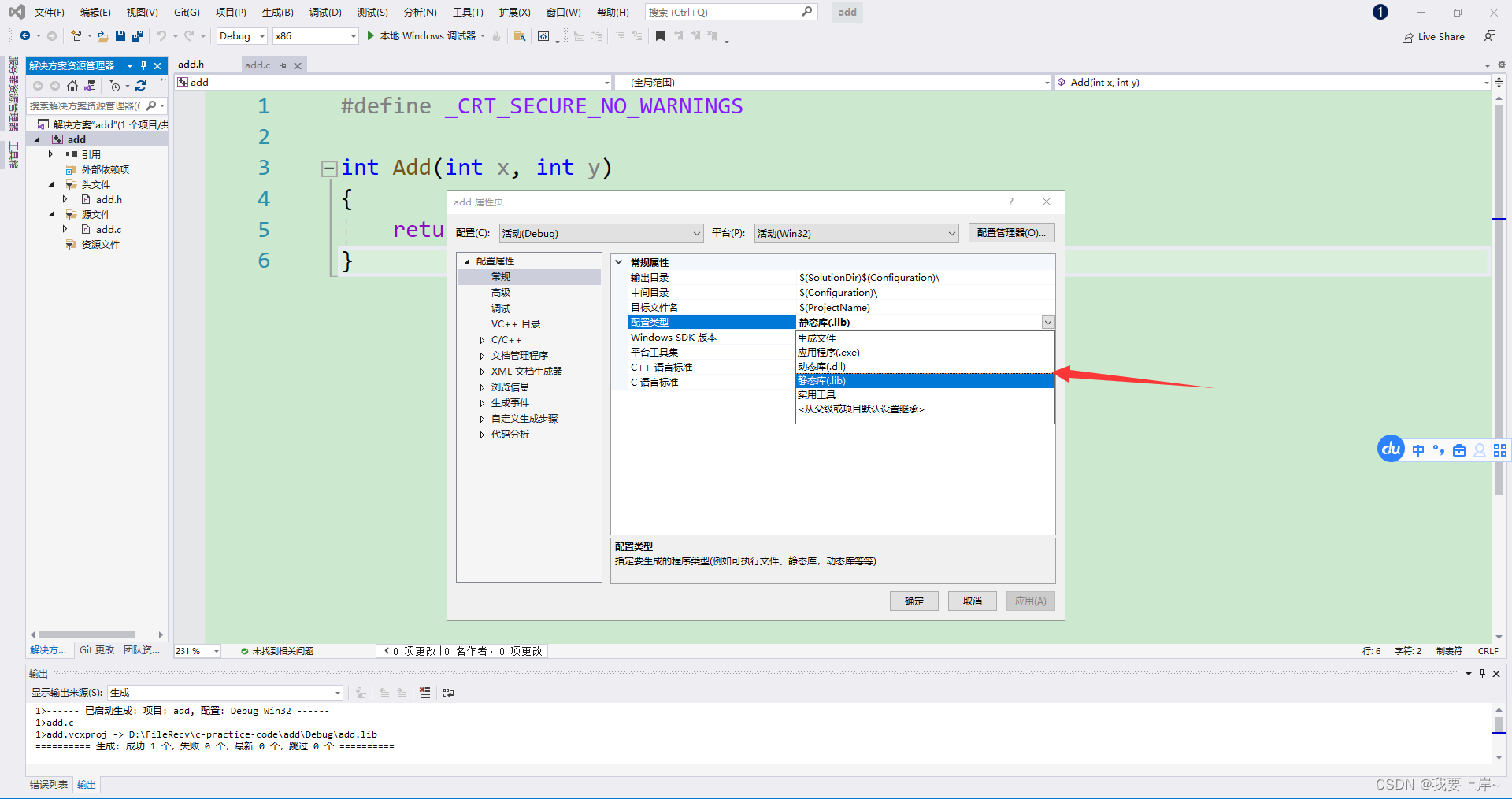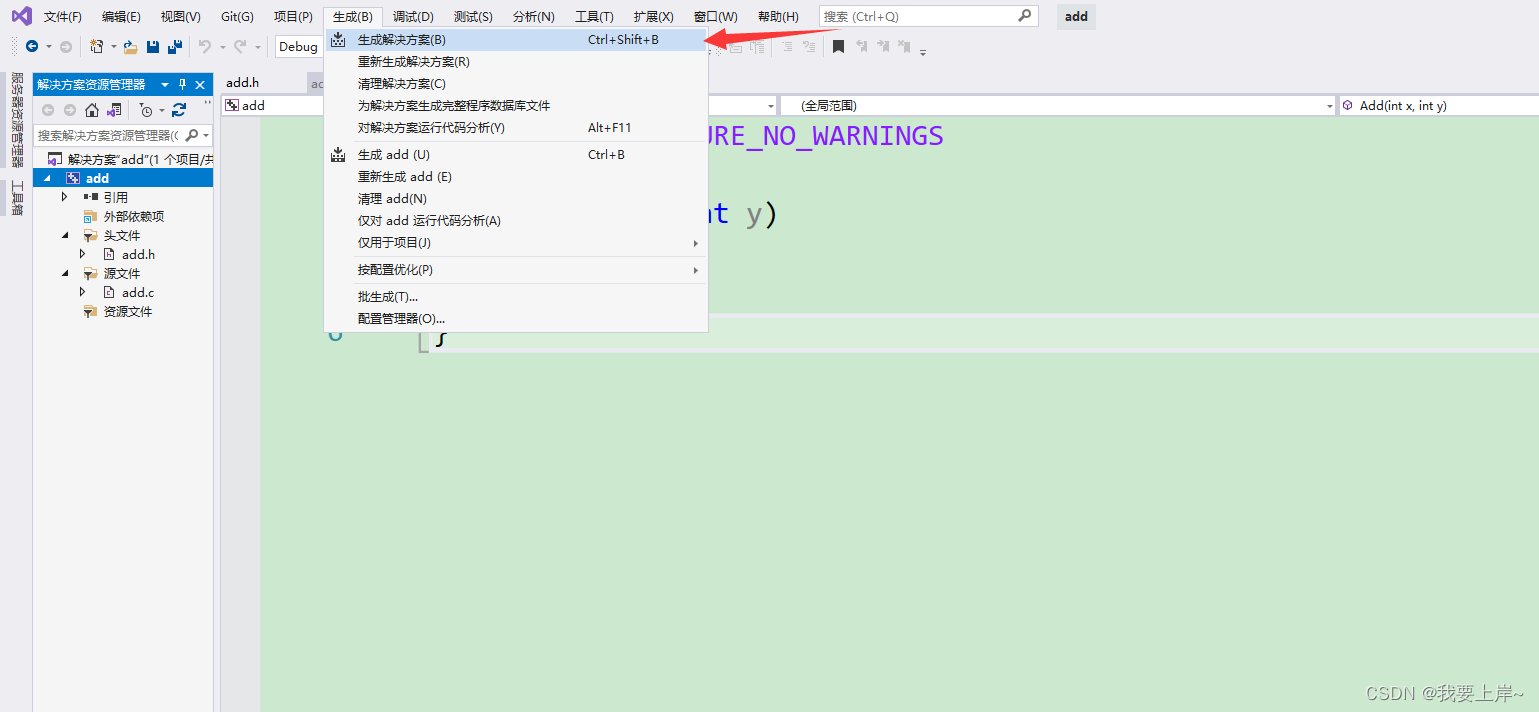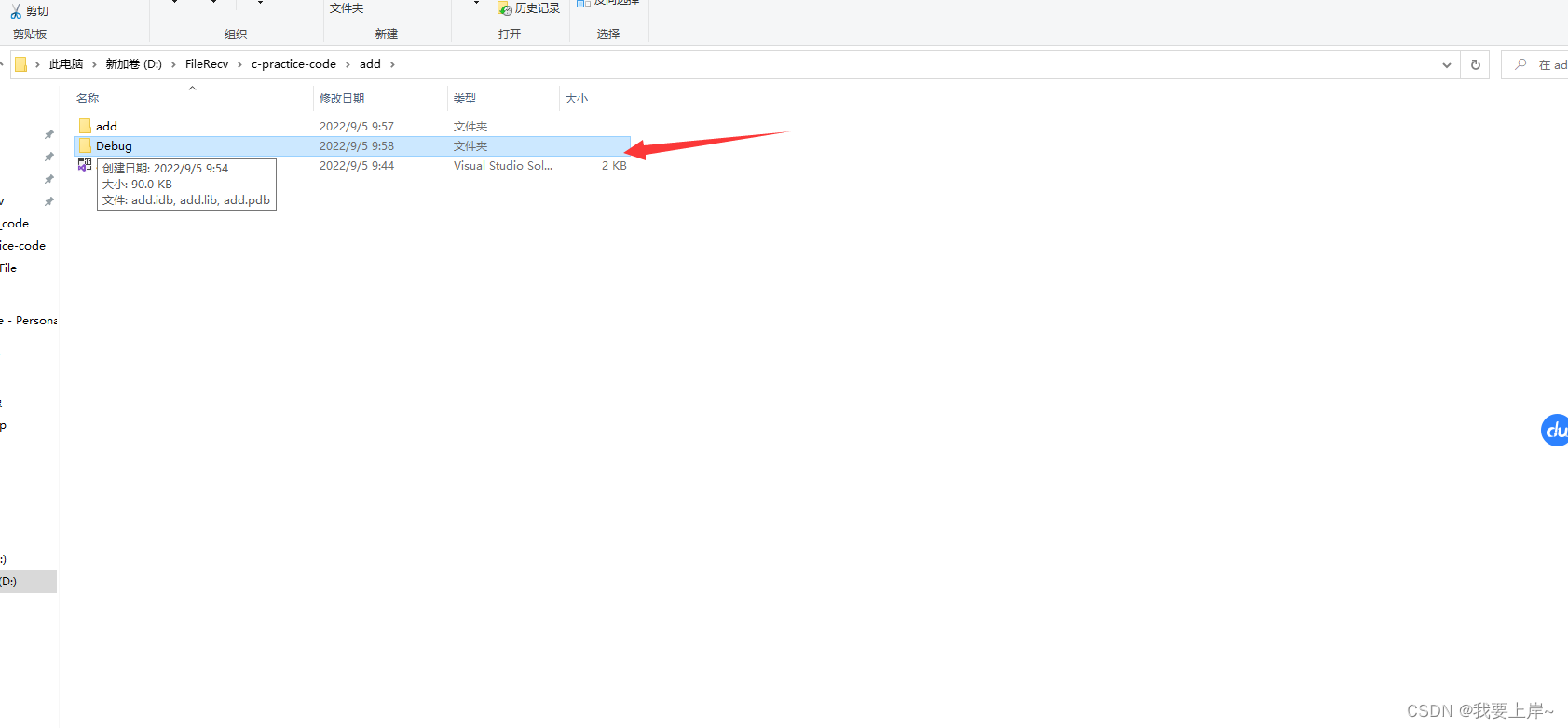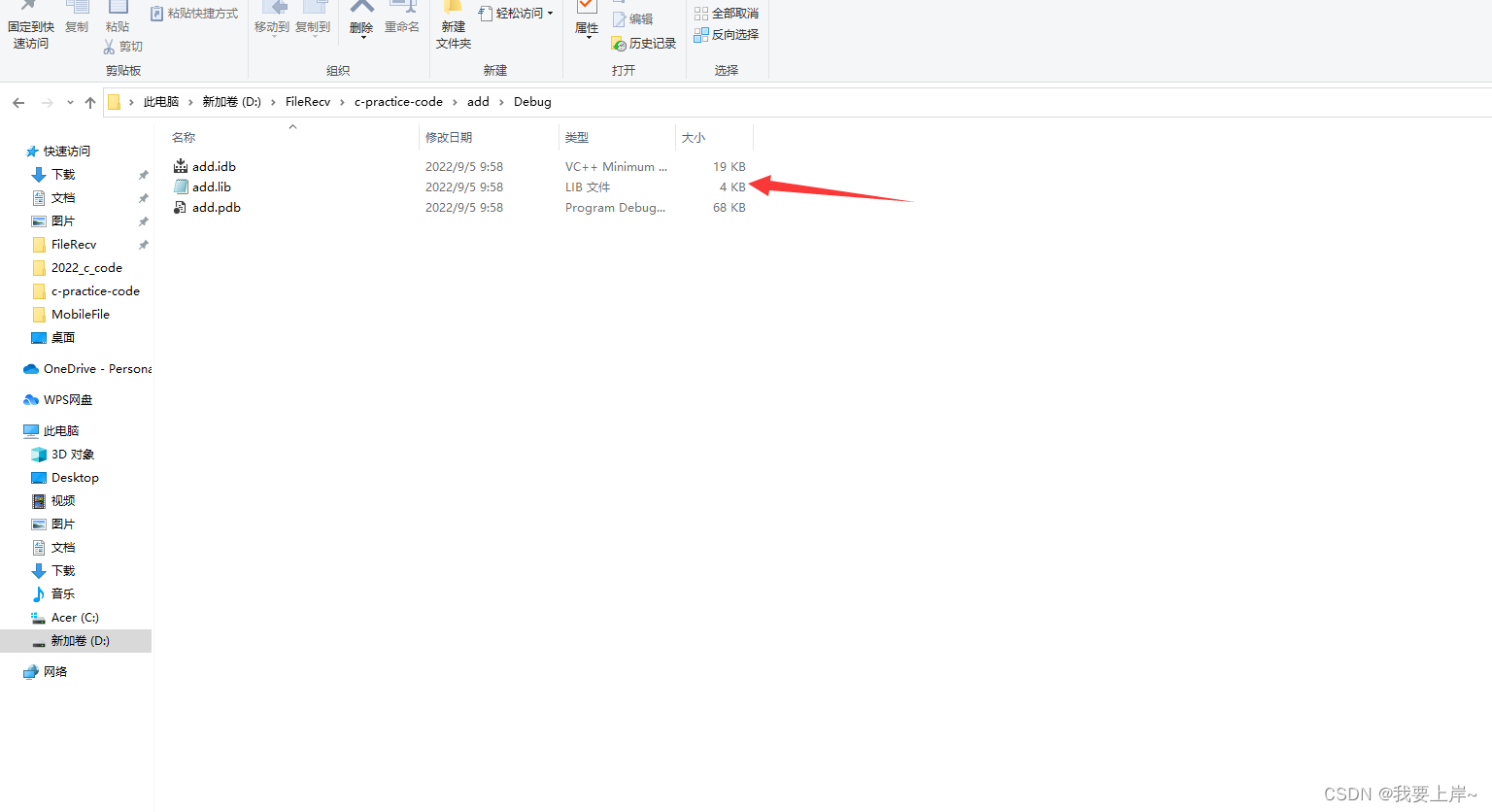用记事本打开add.lib文件，会发现全是乱的。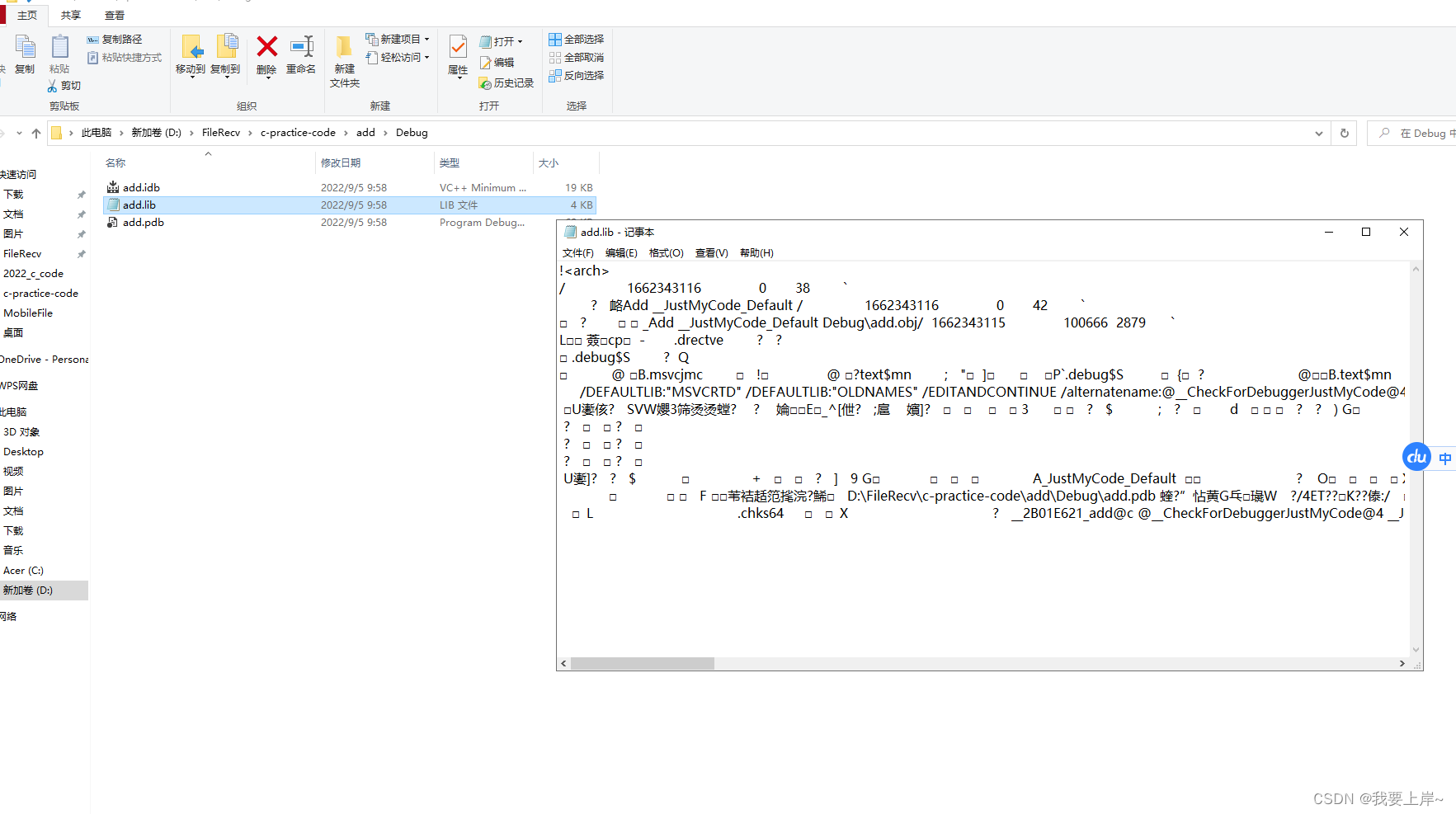然后将add.lib文件 和 add.h文件都放到我们最初的解决方案的文件里面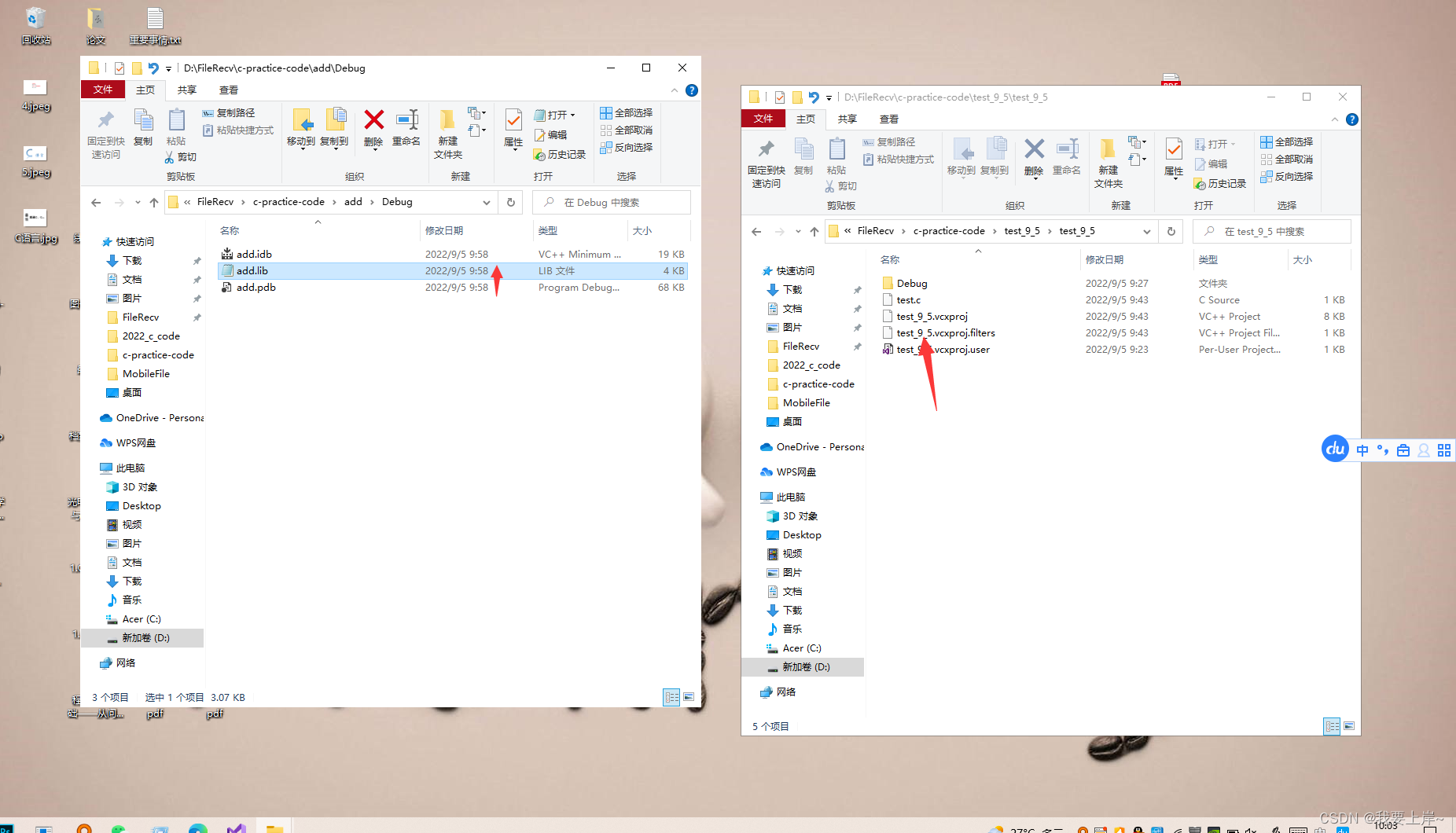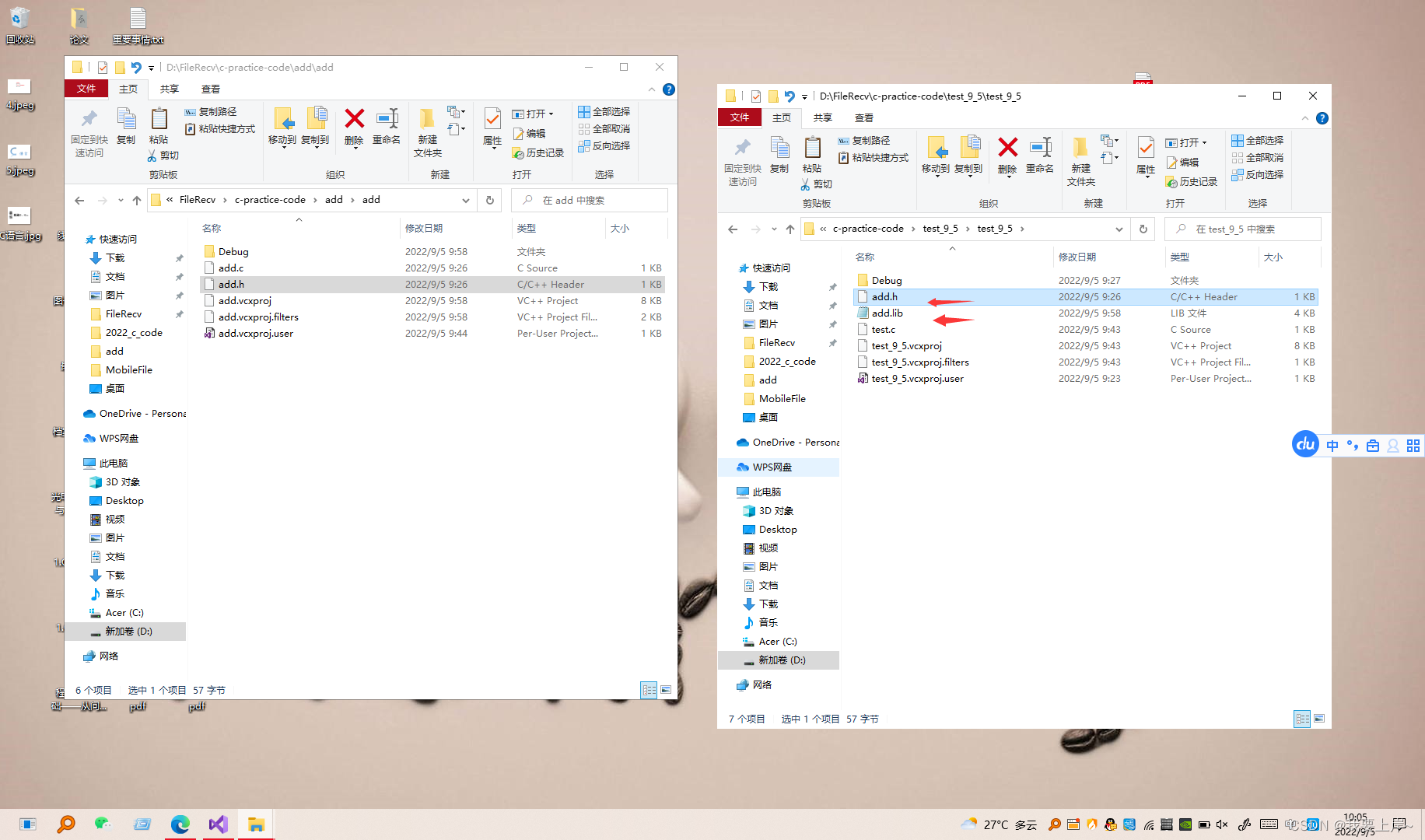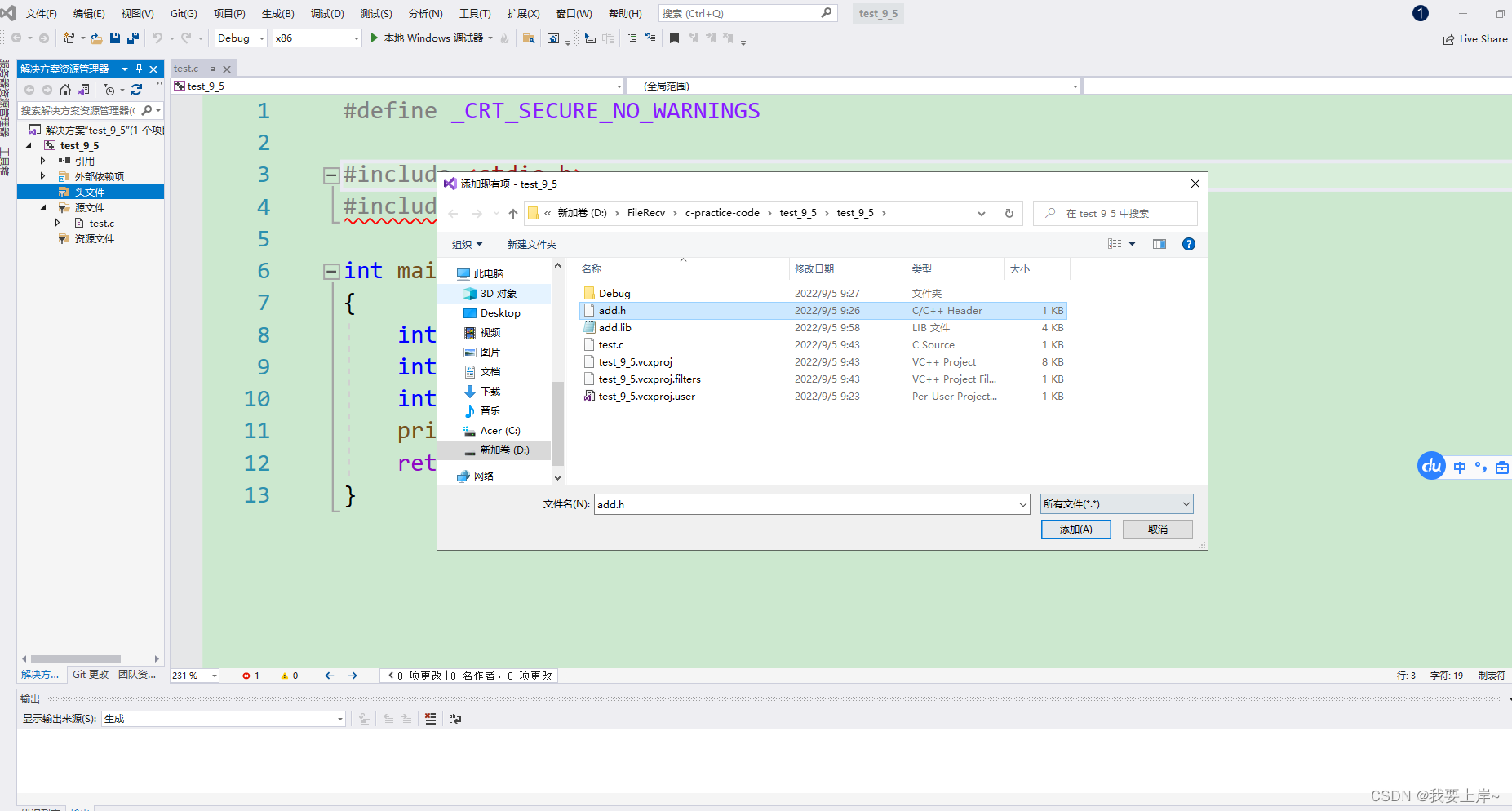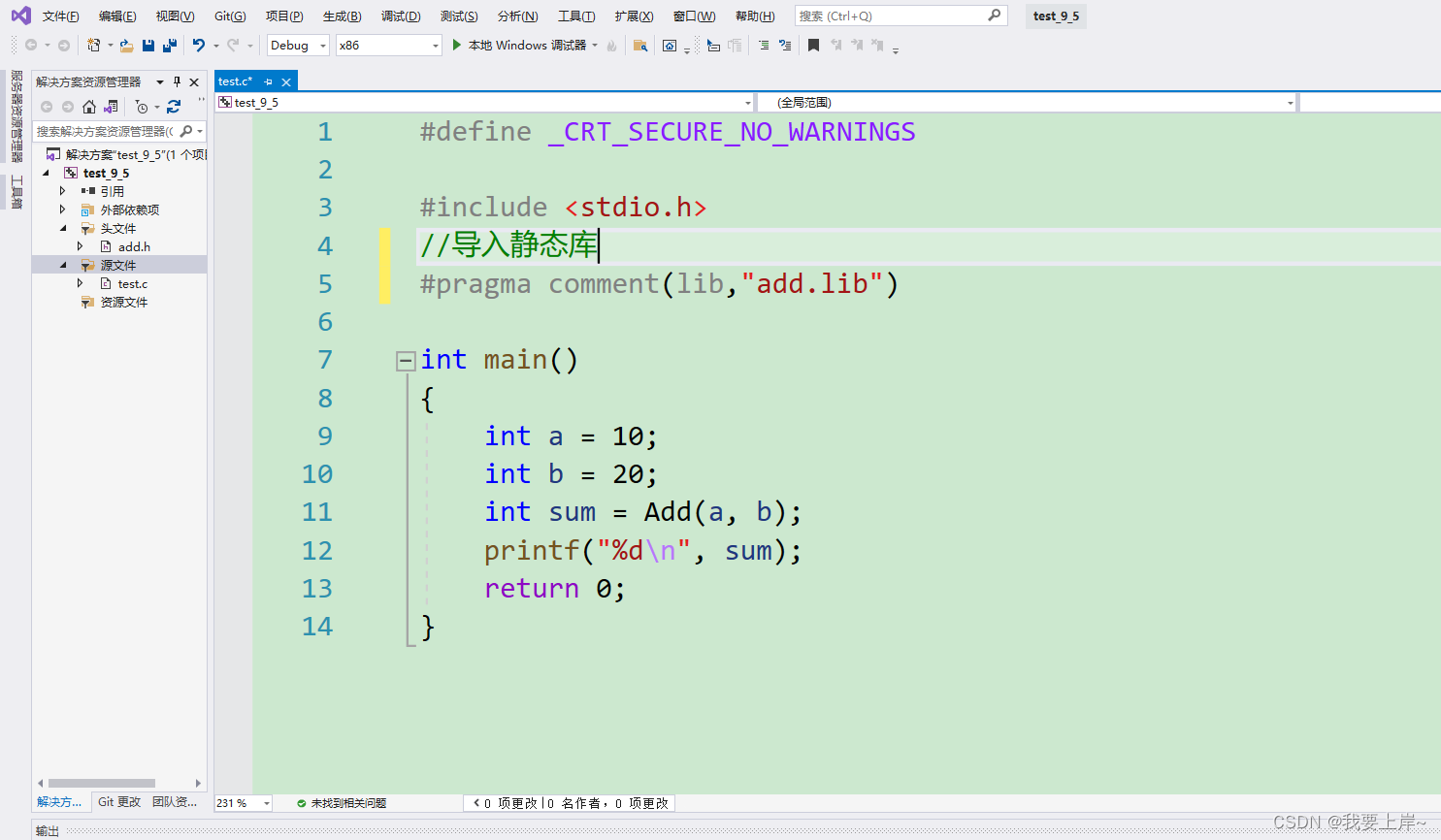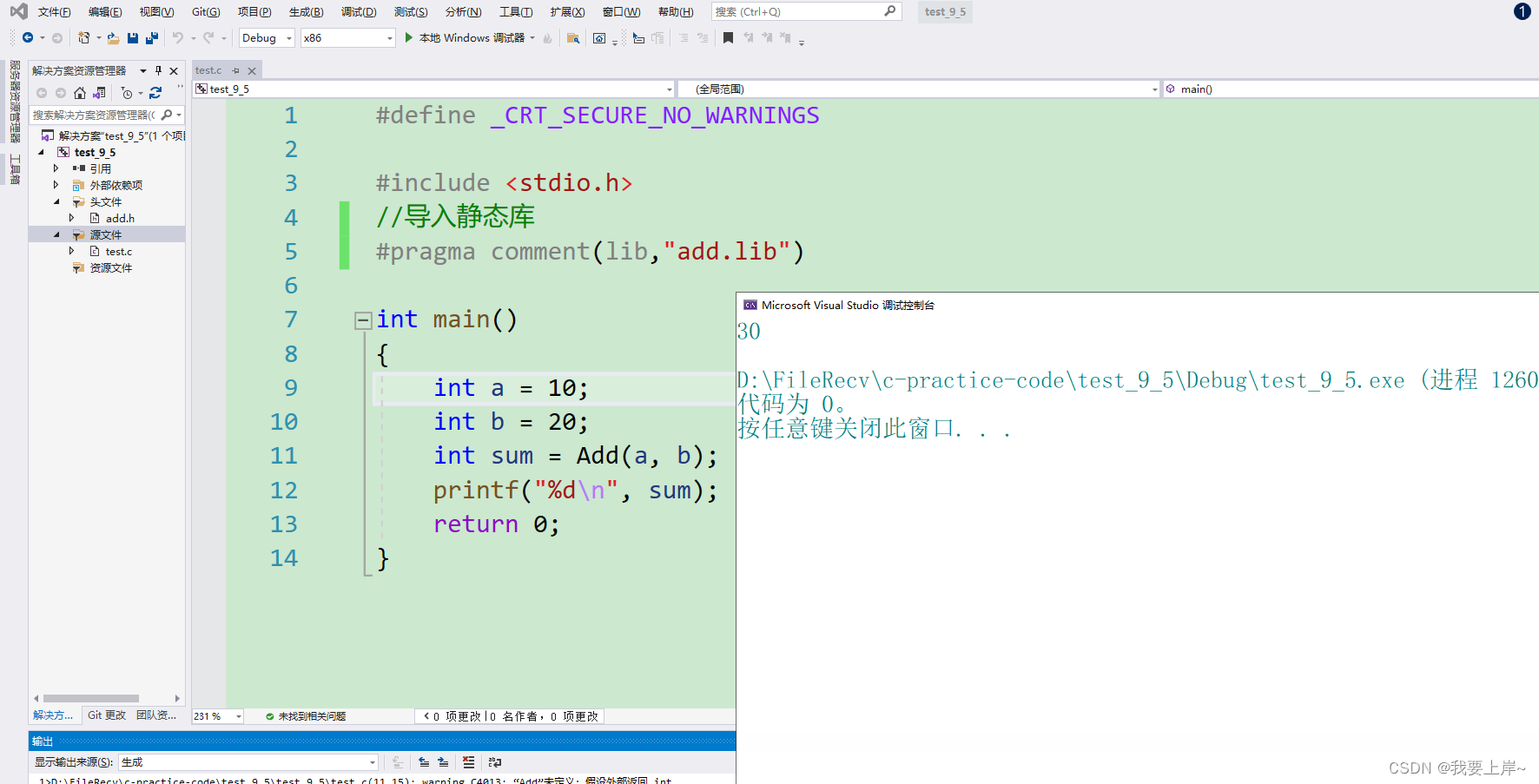# 7. 函数递归

## 7.2 递归的两个必要条件